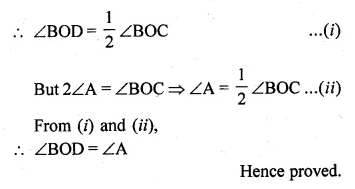Guru

# Ques 11. O is the circumcentre of the triangle ABC and D is mid-point of the base BC. Prove that ∠BOD = ∠A.

• 0

This is the basic and conceptual question from Chapter name- circles
Topic – Angle properties of circles
Chapter number- 15

It is given that O is the circumcentre of the triangle ABC and D is mid-point of the base BC. And we have to Prove that ∠BOD = ∠A by using the properties of circles.

ICSE Avichal publication
Understanding ICSE Mathematics
Question 11

Share